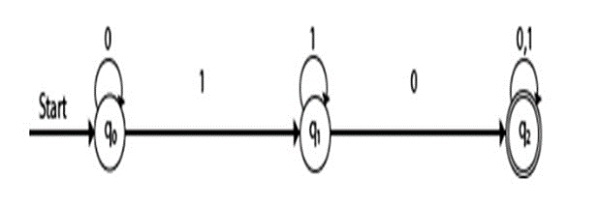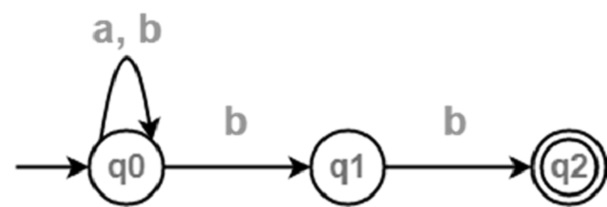# What is the difference between DFA and NFA?

DFA is the short form for the deterministic finite automata and NFA is for the Non-deterministic finite automata. Now, let us understand in detail about these two finite automata.

## DFA

A Deterministic Finite automata is a five-tuple automata. Following is the definition of DFA −

M=(Q, Σ, δ,q0,F)

Where,

• Q : Finite set called states.
• Σ : Finite set called alphabets.
• δ : Q × Σ → Q is the transition function.
• q0 ϵ Q is the start or initial state.
• F : Final or accept state.

## NFA

NFA also has five states same as DFA, but with different transition function, as shown follows −

δ: Q X Σ → 2Q

Where,

• Q : Finite set of states
• Σ : Finite set of the input symbol
• q0 : Initial state
• F : Final state
• δ : Transition function

## Differences

The major differences between the DFA and the NFA are as follows −

Deterministic Finite AutomataNon-Deterministic Finite Automata
Each transition leads to exactly one state called as deterministicA transition leads to a subset of states i.e. some transitions can be non-deterministic.
Accepts input if the last state is in FinalAccepts input if one of the last states is in Final.
Backtracking is allowed in DFA.Backtracking is not always possible.
Requires more space.Requires less space.
Empty string transitions are not seen in DFA.Permits empty string transition.
For a given state, on a given input we reach a deterministic and unique state.For a given state, on a given input we reach more than one state.
DFA is a subset of NFA.Need to convert NFA to DFA in the design of a compiler.
δ : Q × Σ → Q
For example − δ(q0,a)={q1}
δ : Q × Σ → 2Q
For example − δ(q0,a)={q1,q2}
DFA is more difficult to construct.NFA is easier to construct.
DFA is understood as one machine.
NFA is understood as multiple small machines computing at the same time.